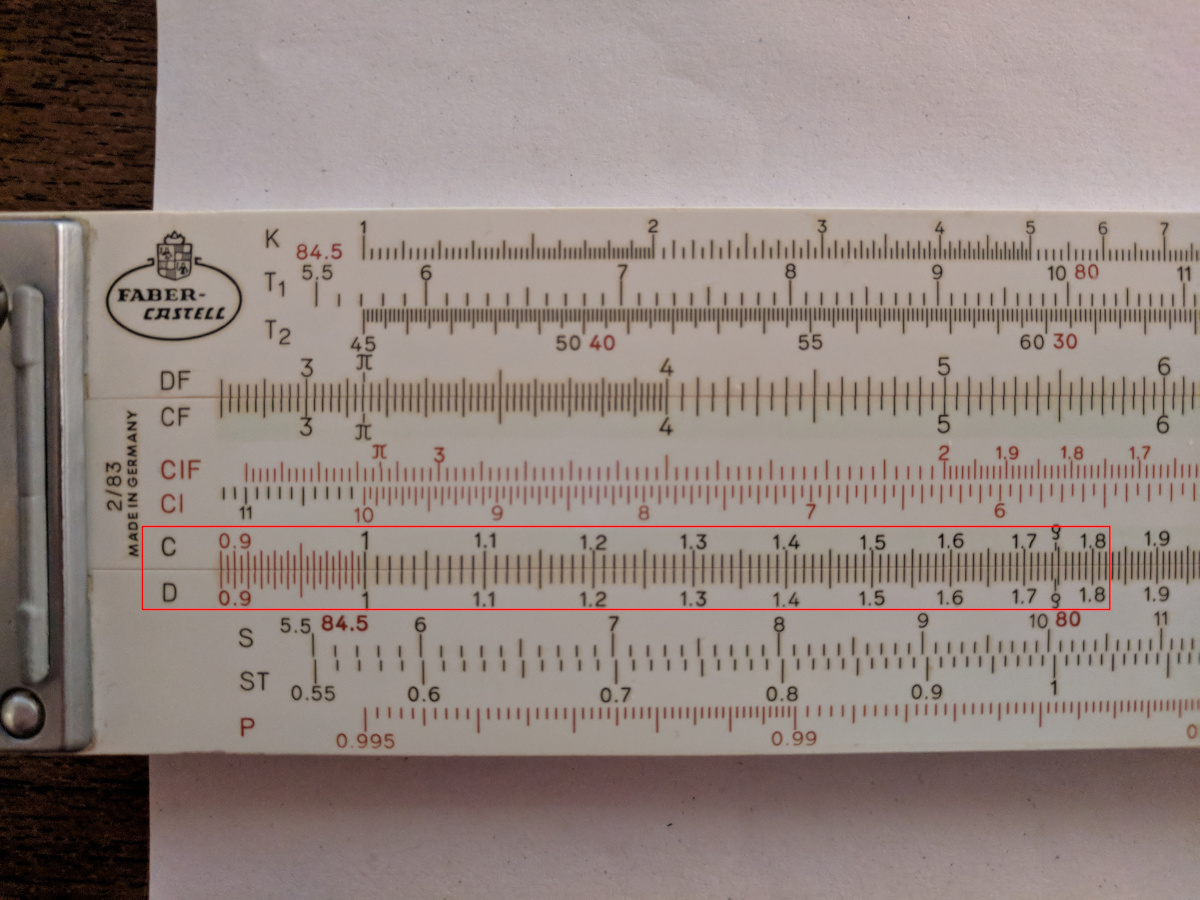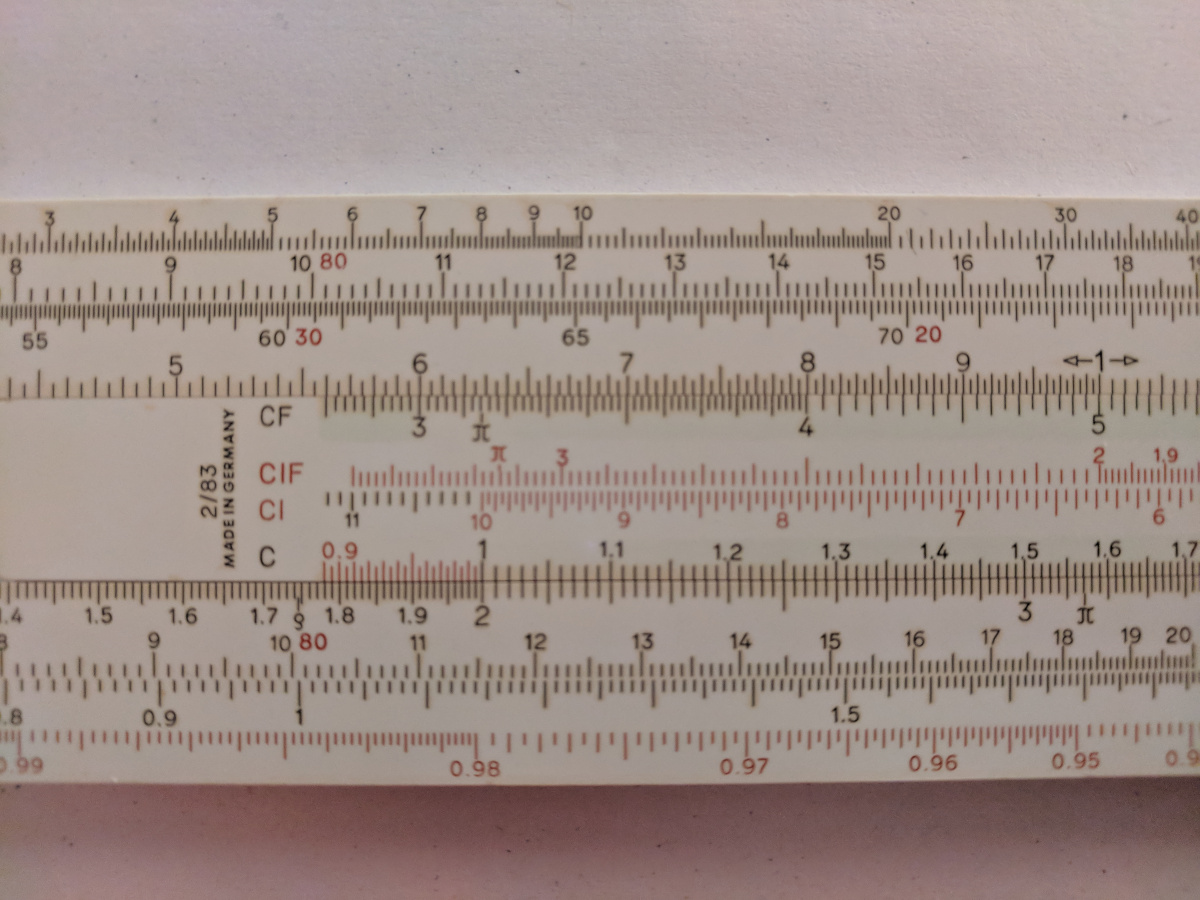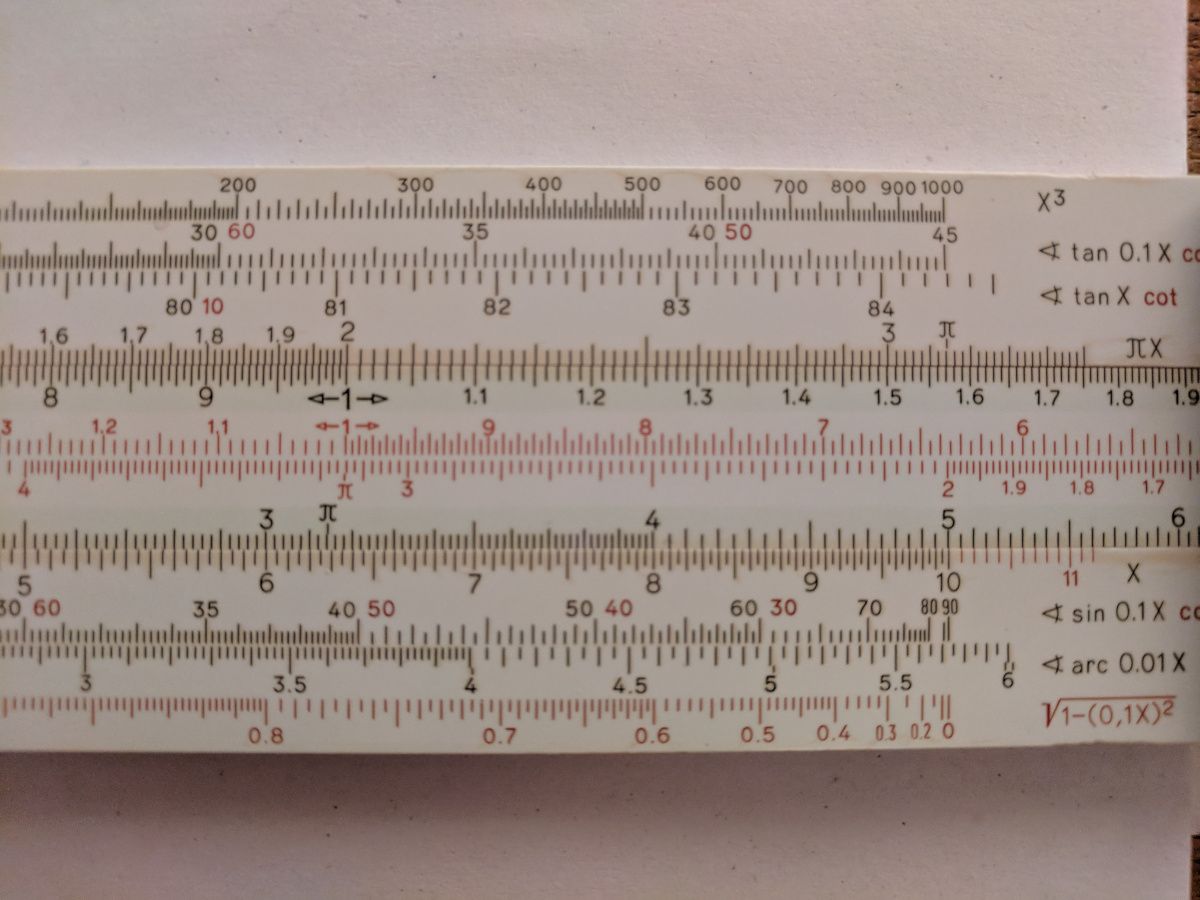# More on slide rules and complex multiplication

Mathematical YouTube channel 3Blue1Brown recently released a video about quaternions, a 4-dimensional extension of the complex numbers now mostly used in 3d graphics and other areas. In the spirit of Edwin A. Abbott, the video builds up to explaining 4d rotations to a 3-dimensional audience by analogy to the step from one dimension to two.

I’d certainly recommend watching the video, as the visualisations are as excellent as they usually are. One of the first ones we see is to explain complex multiplication to a one-dimensional Linelander, starting at 5:12. Seeing it immediately made me think of how multiplication is performed on a slide rule.

Let’s start by going over in detail the process to multiply two numbers, say 2 and 4, with a slide rule. To begin with, here are the two scales that we care about:They are graduated with exactly the same scale, and all of the values line up. This starting position can be thought of as the process ‘multiply by 1’. Since in this example we want to multiply by 2, we slide the movable middle section (scale C) until the 1 lines up with 2 on the lower section (scale D).Now the rule represents the operation ‘multiply by 2’. We can already see everything lines up as you’d expect: already visible is $$2 \times 1.5 = 3$$, and it’s just a matter of looking further along to read off the answer $$2 \times 4 = 8$$.Compare this to the animation in the video. Instead of two adjacent linear scales representing the real line, we have two overlapping coordinate systems for the complex plane. To multiply by $$2 + 3i$$, we move the point 1 on the first grid to the point $$2 + 3i$$ on the second by stetching and rotating it. Then we can read off the product $$(2 + 3i)(1-i)$$ by finding the point on the second grid where $$1 - i$$ ended up.

This process is so similar to how we do it on the slide rule that it really makes me wonder how to build a physical implementation: a complex-valued slide rule. I’m not very practically minded, but I wonder whether there’s something you can do with pantograph linkages like you get in a Hoberman sphere

After a little bit of online searching, I found that of course complex-valued slide rules have been around for a while. The idea of stretching is avoided in a real-valued slide rule by logarithms, and it’s perfectly possible to replicate this in the complex case. All of the examples in the links use the full-blown complex logarithm function; they tend to be cylindrical to handle the rotations gracefully. I particularly recommend looking at the ray-traced image from the third above link. The gently but precisely curving lines and the way the transparent part overlaps the fixed cylinder are both fascinatingly beautiful to look at.